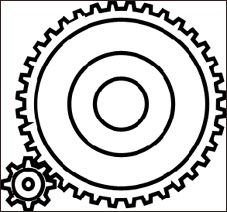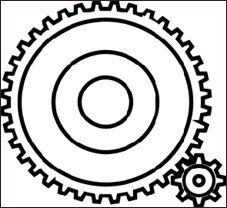Simple Gear Trains

Gearing Down

If the input gear has less teeth than the output gear
the output gear shaft turns more slowly than the
input shaft.

There are three times as many teeth on the
output gear as the input gear.So the input gear
shaft has to turn three times to make the output
gear shaft turn once. The output gear shaft
turns at one third of the input gear shaft.
But with greater force.

This is called GEARING DOWN.

If the input speed on the input gear shaft
is 60 r.p.m what would be the output
speed on the output gear shaft?

A= 20 r.p.mThe small Gear is the Input Gear. The large Gear is the Output Gear.

Gearing Up

If the input gear has more teeth than the output gear
the output gear shaft turns faster than the
input shaft.
There are three times as many teeth on the input
gear as the ouput gear.So the input gear shaft
has to turn once to make the output
gear shaft turn three times. The output gear
shaft turns at three times the speed
of the input gear shaft.
But with greater force.

This is called GEARING UP.

If the input speed on the input gear shaft
is 20 r.p.m what would be the output
speed on the output gear shaft?

B= 60 r.p.mThe large Gear is the Input Gear. The small Gear is the Output Gear.